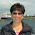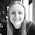## 21 August 2016

### Multiplying Negatives

"A negative times a negative is a positive". It's a hard one to explain. We all learnt it at school and practised it to the point of fluency, but it's not until we're asked why it works that we stop and think about it.

Numbers lines and visualisations are very helpful when teaching the addition and subtraction of negative numbers. But with multiplication and division it's not so clear.

Let's look at a few approaches and resources.

1 . Pattern Spotting
Draw a standard multiplication table and extend it backwards to include negative numbers. It's a straightforward pattern that all students should be able to spot and continue. Get students to do this using Colin Foster's activity on page 5 of his Negative Numbers chapter.
2. Multiplication Grids
Take two 2-digit numbers and multiply them together using grid multiplication. For simplicity, let's take 12 x 11:
Here we have written 12 as 10 + 2 and 11 as 10 + 1. But it would work just as well if we expressed those numbers differently. Instead, let's write 12 as 15 - 3 and 11 as 15 - 4. We should get the same answer:
This only works if -3 x -4 = 12.

Note that this explanation requires students to first understand that positive x negative = negative. This is relatively straightforward to explain in terms of repeated addition.

3. Proof
Here's a proof that is clear and accessible to us experienced mathematicians. I'm not sure how accessible it is to Year 7 students, but it's worth a go.
a and b are positive
a + (-a) = 0
[a +(-a)]•b = 0•b
a•b + (-a)•b = 0
a•b is positive. Therefore (-a)•b is negative

b + (-b) = 0
(-a)•[b + (-b)] = (-a)•0
(-a)•b + (-a)•(-b) = 0
Since (-a)•b is negative, we conclude that (-a)•(-b) is positive.

Perhaps start with a numerical example instead of a formal proof.
3 + (-3) = 0
Multiply everything by -4
3(-4) + (-3)(-4) = 0(-4)
-12 + (-3)(-4) = 0
(-3)(-4) must equal 12 to make this statement true.

It's a good idea to read about a topic before you teach it, even relatively simple topics that you've taught many times before. Here are some helpful links:

I like this clip from Stand and Deliver:

The wording here is important. 'A negative times a negative equals a positive' is clearly preferable to 'two minuses make a plus'. The latter is confusing and may lead to misconceptions. An example of a common mistake is shown below (taken from mathmistakes.org via Nix the Tricks).
Here are a few resource recommendations for this topic:

Colin Foster suggests that you ask students to make up ten multiplications and ten divisions each giving an answer of –8 (eg –2 × –2 × –2 or –1 × 8 etc).

The squaring and cubing (etc) of negatives is worth discussing - students should spot that an even power gives a positive value (eg what is the value of (-1)100?).

It may be worth exploring calculator behaviour too (ie some calculators require brackets when squaring a negative). It's important that students know how to use their calculator properly. There's a great resource from MathsPad for this - Using a Calculator: Odd One Out.

This topic is revisited in later years when students are practising substitution. For example, if a = 3, b = -2 and c = -5, find the values of: abc; bc2; (bc)2; a2b3 and so on. This Substitution Puzzle from mathsteaching.wordpress.com gets quite challenging.

Do let me know if you use an interesting method or resource for teaching the multiplication of negative numbers.

"Minus times minus results in a plus,
The reason for this, we needn't discuss"
- Ogden Nash

1.I sometimes use car depreciation. I show a table of a car's value, decreasing at 2000 per year. (Yes, I tell 'em life isn't that simple.)
If I want to know how much its value has changed I can multiply the number of years by 2000. If I go bakc in time, that would be negative time -- and of course it would be worth more
This doesn't stand a *chance* unless I have the table ready... the visual part of it seems to be important.
The other analogy I make is starting w/ positive times negative being negative (via repeated addition)... and that multiplication is powerful enough to impose its nature on the whole problem, throwing it into reverse. If the other factor is negative... it reverses it again. The "Reverse -- reverse!!" idea works for some and happily doesn't often turn into "-7 + -4 = -11", I think because of the visual difference (there's a plus sign between the "reverse! Reverse!")
MOst of my students have been trhough this stuff a few times and so it's far, far more common for them to do the "two negatives make a positive" thing. I do my best to counter wiht "but two wrongs don't make a right!"

2.I talk about how (-1) means "the opposite" (as in the opposite of 8 is -8 translates in math to be (-1)(8)=8). So using the property of -1 and the community property you could rewrite (-5)(-4) as (-1)(5)(-1)(4) then that can rearrange to (-1)(-1)(5)(4). So since "the opposite of a negative is a positive", that means (-1)(-1)=1, and then 5(4) =20. After we do that a few times we don't need to rewrite the problems, the kids can see that (-6)(-3) would be the opposite of a negative, or a positive 18.

1.Wow I am gonna try this out - thanks for this.

3.Thank you both for these comments, really helpful

4.Thanks for the great post, food for thought for Mondays lesson.

I like to ask pupils what it means if I say you must shout out the answer to this question. (+)

Then you must not shout out the answer...that's +1*-1=-1

Then you must not, not shout out the answer... that translates to -1 * -1 = +1.

A nice logic puzzle that links to language and links back to maths... can lead to some chaotic shouting out though!

5.The odd one out worksheet is fantastic. Good point about the need for practice using a calculator. A lot of intervention done on this skill to date and your post is much appreciated.

1.Thanks, I'm really glad it's useful.